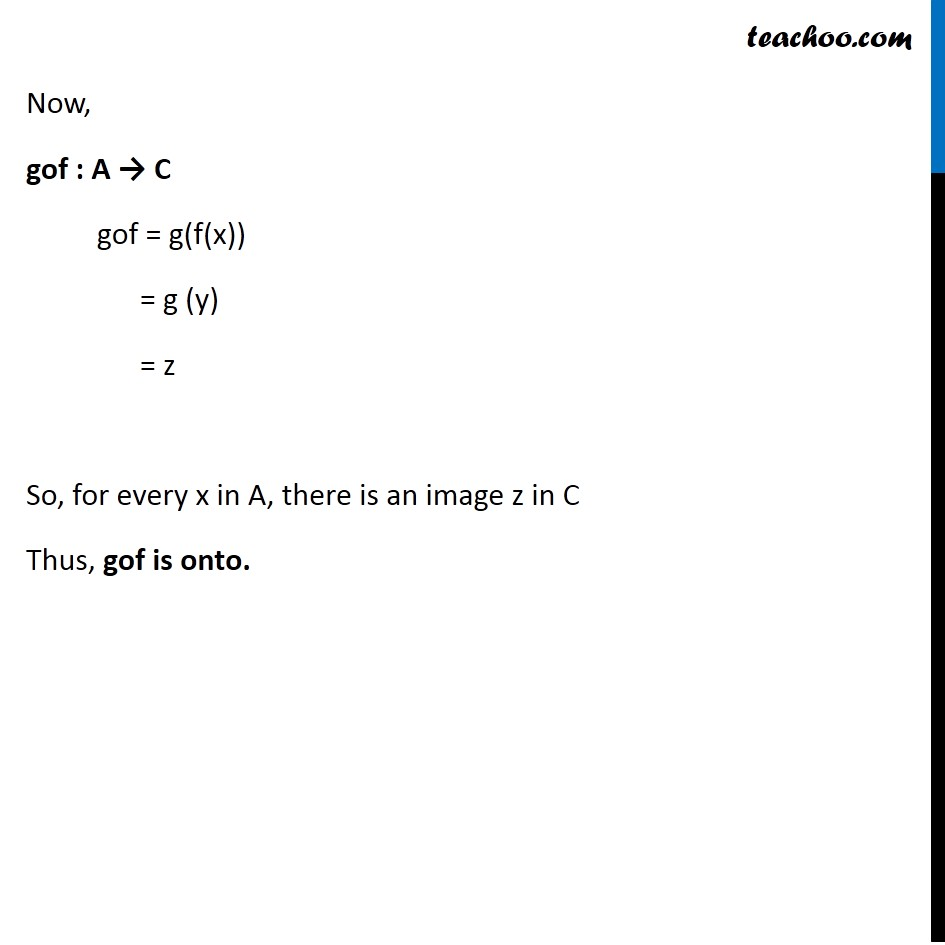Examples

Chapter 1 Class 12 Relation and Functions
Serial order wiseLearn in your speed, with individual attention - Teachoo Maths 1-on-1 Class

### Transcript

Question 3 Show that if f : A → B and g : B → C are onto, then gof : A → C is also onto. Since g : B → C is onto Suppose z ∈ C, then there exists a pre-image in B Let the pre-image be y Hence, y ∈ B such that g (y) = z Similarly, since f : A → B is onto If y ∈ B, then there exists a pre-image in A Let the pre-image be x Hence, x ∈ A such that f(x) = y Now, gof : A → C gof = g(f(x)) = g (y) = z So, for every x in A, there is an image z in C Thus, gof is onto.